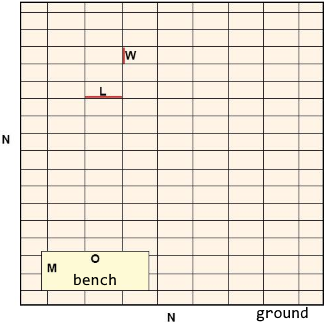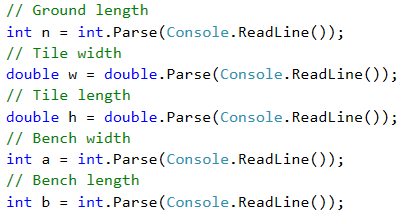# Problem: Change Tiles

The tiles on the ground in front of an apartment building need changing. The ground has a square shape with side of N meters. The tiles are "W" meters wide and "L" meters long. There is one bench on the ground with width of "M" meters and length of "O" meters. The tiles under it do not need to be replaced. Each tile is replaced for 0.2 minutes.

Write a program that reads the size of the ground, the tiles and the bench from the console, and calculates how many tiles are needed to cover the ground and what is the total time for replacing the tiles.

Example: ground with size 20 $m$ has area of 400 $m^2$. A bench that is 1 $m$ wide and 2 $m$ long, has area of 2 $m^2$. One tile is 5 $m$ wide and 4 $m$ long and has area of 20 $m^2$. The space that needs to be covered is 400 - 2 = 398 $m^2$. Therefore, 398 / 20 = 19.90 tiles are necessary. The time needed is 19.90 * 0.2 = 3.98 minutes.

## Input Data

The input data comes as 5 numbers, which are read from the console:

• N – length of a side of the ground within the range of [1 … 100].
• W – width per tile within the range of [0.1 … 10.00].
• L – length per tile within the range of [0.1 … 10.00].
• М – width of the bench within the range of [0 … 10].
• О – length of the bench within the range of [0 … 10].

## Output Data

Print on the console two numbers: number of tiles needed for the repair and the total time for changing them, each on a new line.

## Sample Input and Output

Input Output
20
5
4
1
2
19.9
3.98

Explanation of the example:

• Total area = 20 * 20 = 400.
• Area of the bench = 1 * 2 = 2.
• Area for covering = 400 - 2 = 398.
• Area of tiles = 5 * 4 = 20.
• Needed tiles = 398 / 20 = 19.9.
• Needed time = 19.9 * 0.2 = 3.98.
Input Output
40
0.8
0.6
3
5
3302.08333333333
660.416666666667

## Hints and Guidelines

Let's make a draft to clarify the task requirements. It can look the following way:### Idea for Solution

It is required to calculate the number of tiles that have to be changed, as well as the total time for replacing them. In order to find the number of tiles, we have to calculate the area that needs to be covered and divide it by the area per tile.The ground is square, therefore, we find the total area by multiplying its side by its value N * N. After that, we calculate the area that the bench takes up by multiplying its two sides as well M * O. After subtracting the area of the bench from the area of the whole ground, we obtain the area that needs to be repaired.

We calculate the area of a single tile by multiplying its two sides with one another W * L. As we already stated, now we have to divide the area for covering by the area of a single tile. This way, we find the number of necessary tiles which we multiply by 0.2 (the time needed for changing a tile). Now, we have the wanted output.

### Choosing Data Types

The length of the side of the ground, the width and the length of the bench, will be given as integers, therefore, in order to store their values, we can declare variables of int type. We will be given floating-point numbers for the width and the length of the tiles and this is why we will use double. The output will be a floating-point number as well, so the variables will be of double type as well.

### Reading the Input Data

As in the previous tasks, we can divide the solution into three smaller tasks:

• Reading the input from the console.
• Doing the calculations.
• Printing the output on the console.

The first thing we have to do is go through the input of the task. It is important to pay attention to the sequence they are given in. With Console.ReadLine(…) we read values from the console and with int.Parse(…) and double.Parse(…), we convert the particular string value into int or double.### Performing the Calculations

After we have initialized the variables and have stored the corresponding values in them, we move to the calculations. As the values of the variables n, a and b are stored in variables of type int, we can also declare variables of the same type for the results.The variables w and h are of type double, therefore, for the area of a single tile, we create a variable of the same type. Finally, we calculate the values that we have to print on the console. The number of necessary tiles is obtained by dividing the area that needs to be covered by the area of a tile. When dividing the two numbers, one of which is a floating-point number, the result will also be a floating-point number. Therefore, in order for the calculations to be correct, we store the result in a variable of type double. The task does not specify special formatting or rounding of the output, so we just print the values with Console.WriteLine(…).## Testing in the Judge System

Test your solution here: https://judge.softuni.org/Contests/Practice/Index/505#2.